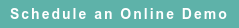# RoyaltyStat Blog

### Posts by Topic

An autoregressive (AR) model can produce more reliable measures of comparable company profit ratios (operating margin or profit rate) than the naive profit model prescribed by the OECD transfer pricing guidelines. We prefer to work with profit margins because they are pure numbers, unlike profit rates over assets of different vintages. Here, we show a fixed-point equilibrium and variance of an AR(1) model allowing the computation of a comparable profit ratio interval to benchmark related party transfers of goods and services. This AR(1) model can be used also to benchmark routine functions (manufacturing, distribution, retail) under the residual profit split method.

Fixed-Point Equilibrium

Consider a simple AR(1) model of company profit ratios:

(1)     Y(t + 1) = α + β Y(t) + U(t),

where Y(t) is stable when the absolute value of the slope coefficient is lower than one (|β| < 1), and the error term U(t) has zero mean and constant variance, σ2.

The variable Y(t) is the profit ratio in year t = 0, 1, 2, 3, …, T of a selected comparable company, and U(t) is a random error because the relationship is stochastic. In transfer pricing, T ≤ 5 years. However, we get more reliable results if we consider more data points than the usual three to five years of company profit ratios. Three years of data are not sacrosanct.

Let μ be the mean (average) operating profit ratio, and E is the expected value operator. The expected value of equation (1) is the simple formula:

(2)     E(Y(t + 1)) = α + β E(Y(t)), where E(U(t)) = 0.

(3)     μ = α + β μ from which we get a fixed-point equilibrium:

(4)     μ = α / (1 − β), which is positive as long as the intercept of (1) is positive.

If α = 0, then μ = 0 and Y(t) fluctuates around zero. See Walter Enders, Applied Econometric Time Series, John Wiley & Sons, 1995, which is a good reference for AR models, including coverage of the Dickey-Fuller test for β = 1 (i.e., the random walk model). A fixed point “is also called a rest point of a flow, or an equilibrium solution of a differential equation. The rates of change vanish at these points and the variables of the differential equation remain constant.” Florin Diacu & Philip Holmes, Celestial Encounters (The Origins of Chaos and Stability), Princeton University Press, 1996, pp. 31, 153, showing the behavior of various flows near a rest point.

Calculating an Equilibrium Profit Margin

As example, the operating profit margin of Target Corp. (CIK 27419; GVKEY 3813) from 1978 to 2016 appears stationary (without a discernible up or down trend during the measured period). Using Compustat®’s acronym for the “operating margin after depreciation” (OMAD), we used OLS (ordinary least squares) regression analysis to obtain the AR(1) estimates:

from which we computed the equilibrium value (using equation (4)):

(4)     μ = 2.037/(1 – 0.696) = 6.7% ± 0.7%

Target Corp.’s equilibrium OMAD is close to the mean = 6.773%, with a small standard deviation = 0.98%, and quartiles are Q1 = 6.276%, Q2 (Median) = 6.911%, and Q3 = 7.432%. The very narrow standard errors and interquartile range (IQR = 1.156%) vitiate our need to compute a confidence interval for Target's equilibrium point. The action proposed here is that we would estimate the intercept (α) and slope (β) coefficients of the AR(1) model for every comparable company to the tested party, and then compute a range of their equilibrium values. We used 39 data points (Year 1 = 1978 to 2016) and the R-square = 49.9%. The t-statistic of the slope coefficient of AR(1) is significant (4.33), but not that of overfitting AR(2) (1.78) to correct for potential serial correlation among the residuals of (1).

Variance and Profit Interval

The variance of Y(t) is computed with the formula (ignoring the covariance between the current and lagged values of the dependent variable):

(6)     V(Y(t + 1)) = β2 V(Y(t)) + σ2,

which is larger than the variance of the random error (σ2) because the term β2 V(Y(t)) is greater than zero.

Using equation (4) and the square root of (6), we can compute a central value (fixed-point equilibrium) and its encompassing interval for the selected operating profit ratio. In RoyaltyStat®, we have a built-in AR(p) function (where the order p = 1 to 5) that clients can combine with our subscription-based Global Compustat company financials online database to select comparables to the tested party and determine a range of equilibrium profit ratios to benchmark controlled crossborder transfers of traded goods and services.

Published on Mar 31, 2017 5:53:43 PM

Ednaldo Silva (Ph.D.) is founder and managing director of RoyaltyStat. He helped draft the US transfer pricing regulations and developed the comparable profits method called TNNM by the OECD. He can be contacted at: esilva@royaltystat.com

RoyaltyStat provides premier online databases of royalty rates extracted from unredacted license agreements
and normalized company financials (income statement, balance sheet, cash flow). We provide high-quality data, built-in analytical tools, customer training and attentive technical support.Topics: Net Profit Indicator